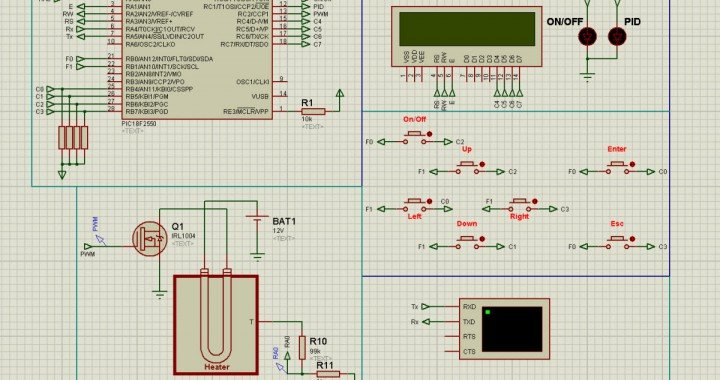# Designing and Simulation of Industrial PID Controllers using Microcontrollers

Ing. Cristoforo Baldoni

In this article we’ll see how to pass from the design of analog PID controllers for continuous-time systems to digital controllers, replacing operational amplifiers, resistors and capacitors with microcontrollers. Digital controllers are very compact, all the controller fits on a chip, including the A/D and the D/A converters, moreover, digital controllers are not affected by the aging of the components and don’t change their values with the temperature as analog components do. We’ll see how to apply the Z-transform, the equivalent of the Laplace transform, but for discrete-time systems, we’ll see how to identify the transfer function of a process and we’ll explain, with a step by step procedure, how to apply the theoretical knowledge learnt by examining an Proteus microcontroller based project, that uses its PWM output to control an oven ‘s temperature. The microcontroller has a 10 bit A/D converter. This procedure can be easily adapted with minimal adjustments to other processes to control.

Topics

1. Digital Control-System Block Diagrams

2. Linear difference equations, Z-Transform, Inverse Z-Transform and Discrete Transfer Function.

3. Sampling and A/D Analogic to Digital Converter

4. D/A Digital to Analogic Converter and ZERO ORDER HOLD  (ZOH) : Relationship between the Continuous Transfer Function and Discrete Transfer Function of a sampled Process.

5.  Block Diagram Manipulation of Sampled Data Systems

6. Methods for designing Digital Controllers, Stability.

7. Designing  PID controllers by microcontrollers

8. Transfer Function Identification and PID Tuning using the Ziegler–Nichols Method.

9. Practical case of a temperature control system implemented with a microcontroller PIC and simulated with ISIS Proteus: Step by step explanation of how to apply the theoretical knowledge for implementing and simulating a PID controller.